# 论文引入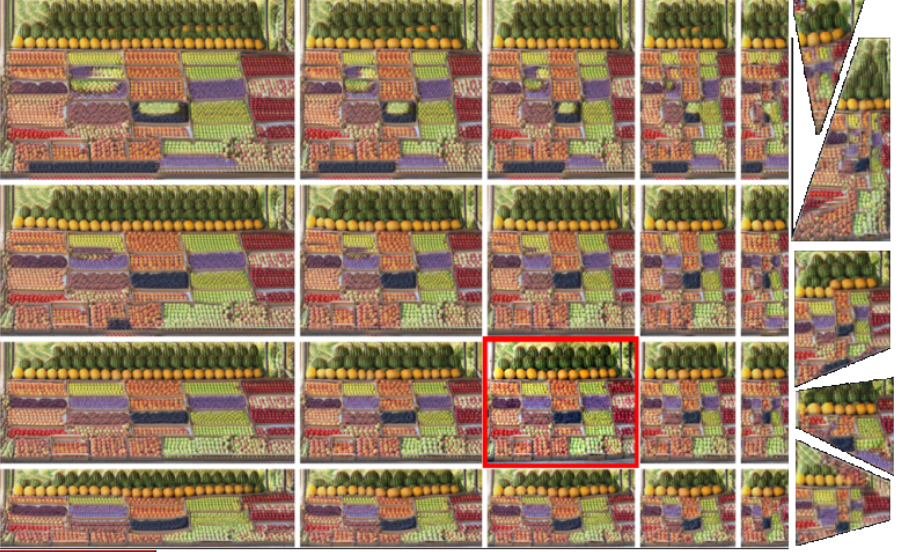• 不仅要求所有输入色块都在输出中，而且还需要保持这些色块的频率相同。
• 通过匹配分布而不是单个补丁。
• 通过已训练的网络进行一次前向遍历可以生成任何大小/形状/长宽比的目标图像。

• InGAN为各种不同的任务和不同的数据类型提供统一的框架，所有这些都具有一个单一的网络体系结构。
• InGAN可以产生大小，形状和纵横比明显不同的输出，包括非矩形输出图像。
• InGAN是第一个在单个自然图像上训练GAN（训练过程中只是对一张图像进行训练和学习）。

# InGAN框架

InGAN是如何训练一张图像达到图像重定向的呢？我们先从整体架构上去理解，图2展示了InGAN的框架：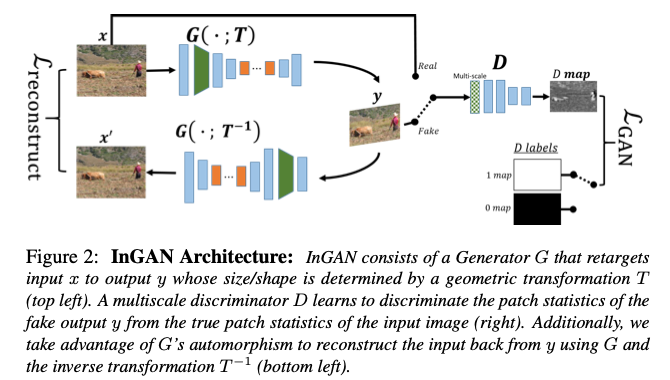$\mathcal L_{GAN}(G,D) = \mathbb E_{x \sim p_{data}(x)}[(D(x)-1)^2]+\mathbb E_{x \sim p_{data}(x)} [D(G(x))^2]$

$\mathcal L_{reconst} = \Vert G(G(x;T);T^{-1})-1 \Vert_1$

$\mathcal L_{InGAN} = \mathcal L_{GAN} + \lambda \cdot \mathcal L_{reconst}$

# 设计细节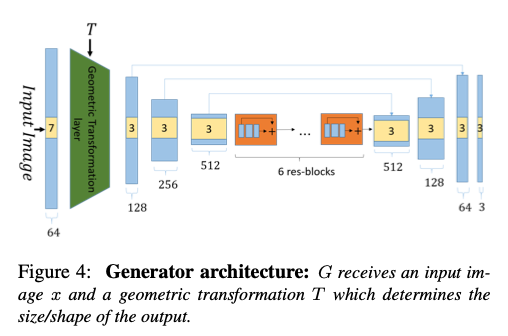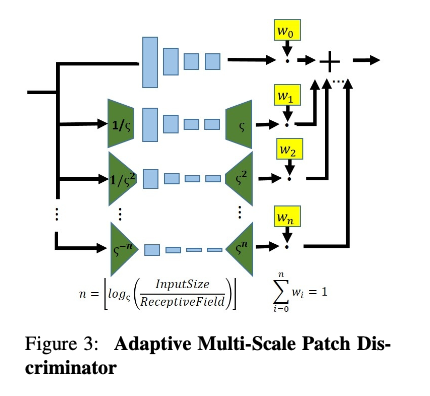# 实验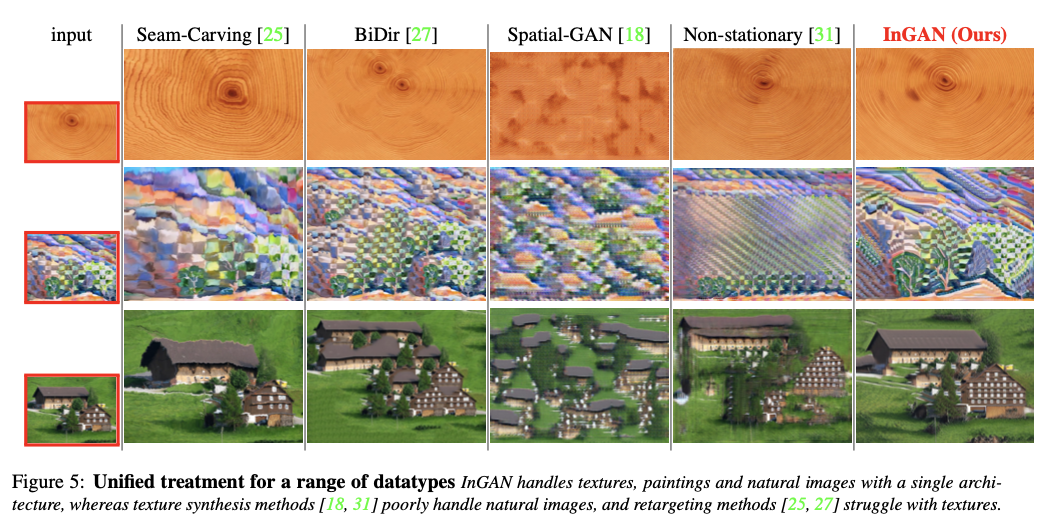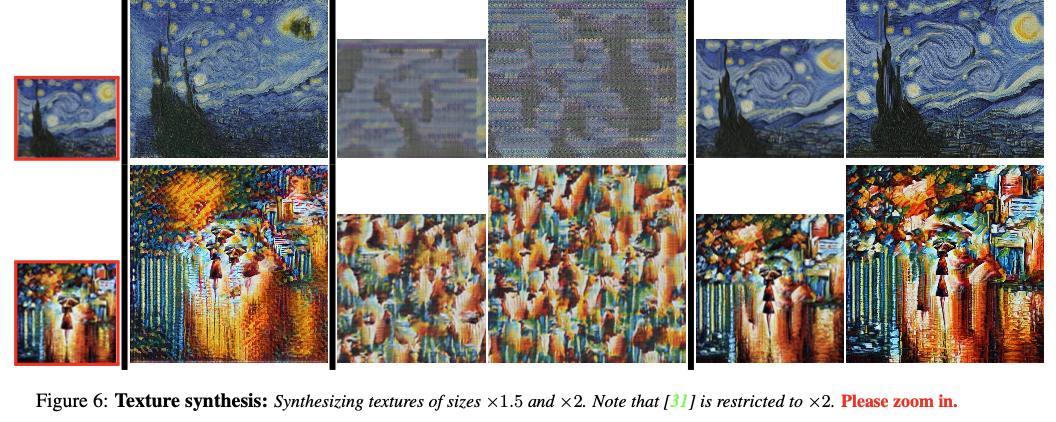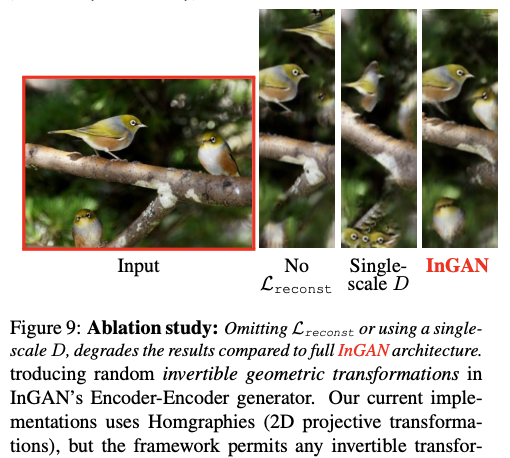# 总结

InGAN通过对单一图像的内部色块分布进行学习和分布匹配，在内部色块被学习到后可以实现图像的自然拉伸和重定向（可以产生大小，形状和纵横比明显不同的输出，包括非矩形输出图像）等任务，同时这个框架便可适用于这些任务而不需要再去更改。

# 参考文献

 A. Buades, B. Coll, and J.-M. Morel. A non-local algorithm for image denoising. In CVPR, volume 2, pages 60–65, 2005. 1

 D. Simakov, Y. Caspi, E. Shechtman, and M. Irani. Summarizing visual data using bidirectional similarity. In The IEEE Conference on Computer Vision and Pattern Recognition (CVPR), 2008. 1, 4, 5, 7

 Zhu J Y, Park T, Isola P, et al. Unpaired image-to-image translation using cycle-consistent adversarial networks[C]//Proceedings of the IEEE international conference on computer vision. 2017: 2223-2232.

 X. Mao, Q. Li, H. Xie, R. Y. K. Lau, and Z. Wang. Least squares generative adversarial networks. In Computer Vision (ICCV), IEEE International Conference on, 2017. 4